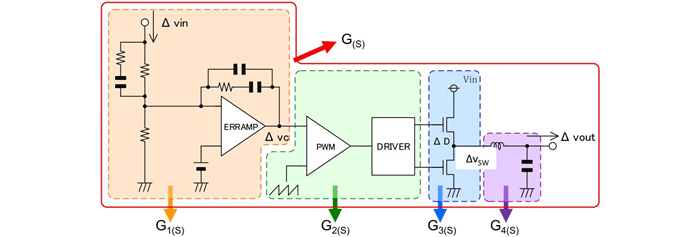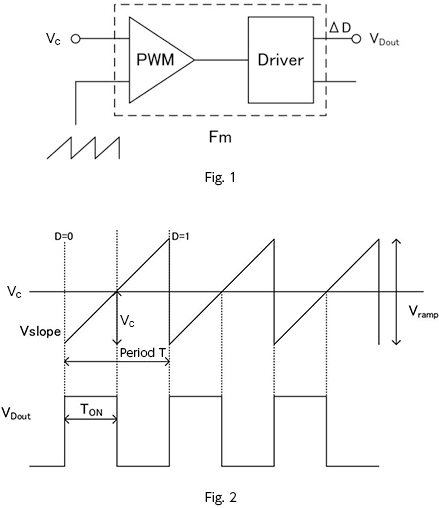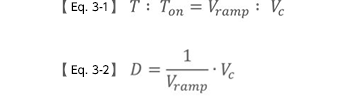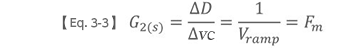Technical Information Site of Power Supply Design

2017.01.12 Transfer Function

# Slope Transfer Functions: Voltage-Mode Transfer Functions

DC/DC Converters: Sharing of Transfer Functions among Control Systems

From here, we consider slope transfer functions. Below is reproduced Fig. 2 from [Basic Approach to Transfer Functions and Their Derivation], but from this section, we will explain the part G2(S), consisting of a PWM component and driver, that follows the error amplifier G1(S) of the previous section. In representative PWM control, there are a voltage mode and a current mode. We begin with transfer functions for the voltage mode.Voltage-mode transfer functions

Voltage-mode control in a DC/DC converter is the most basic system. Only the output voltage is fed back through a feedback loop. By using the voltage difference obtained by comparison with a reference voltage using an error amplifier, and further comparing with a slope wave (triangular wave), the pulse width of a PWM signal is determined and the output voltage is controlled.

Fig. 1 shows a block in which the output Vc from the error amplifier and the slope voltage are input to the PWM generator to generate the PWM output.

Here, by comparing a fixed slope wave with Vc, the transfer function of the block Fm, which is PWM controlling the duty D = Ton/T, is derived.

Fig. 2 shows the input waveform and the PWM output waveform. The slope wave rises with a constant slope by Vramp over one period. Through comparison with Vc, the on and off times of the driver output VDout, that is, the duty cycle, is determined. The control conditions are as follows.

When Vc ＞ Vslope , VDout = ON
When Vc ＜ Vslope , VDout = OFF

Moreover, in Fig. 2:

When Vslope = Vc = ０  ,    D = 0
When Vslope = Vc = Vramp , D = 1

Equation 3-1 is obtained from similarity of the triangle formed from Vslope and Vc, and by modifying this equation using D = Ton/T, we obtain equation 3-2.Moreover, from perturbation of equation 3-2 （ D → D + ∆D , Vc → Vc + ∆VC） equation 3-3 is obtained.